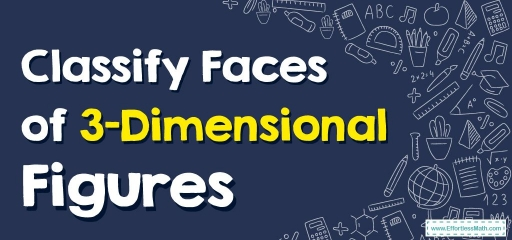# Classify Faces of 3–Dimensional FiguresA $$3D$$ shape is a solid which comprises three dimensions – length, breadth (width), and height. The $$3D$$ in the term $$3D$$ shapes implies $$3$$-dimensional.

All $$3D$$ geometric shapes occupy space dependent on their dimensions and you can notice lots of $$3D$$ shapes everywhere in daily life.

A few cases of $$3D$$ shapes would be cubes, cuboids, cones, and cylinders.

## Characteristics of 3D shapes

$$3D$$ shapes will have faces (sides), as well as edges and vertices (corners).

### Faces

Faces are flat or curved surfaces on a $$3D$$ shape. For instance, cubes have $$6$$ faces, while cylinders have $$3$$ and spheres have only $$1$$.

### Edges

Edge is where $$2$$ faces meeting. For instance, cubes have twelve edges, while cylinders have $$2$$ and spheres don’t have any.

### Vertices

Vertices are corners where some edges link. For instance, cubes have $$8$$ vertices, while cones have $$1$$ vertices and spheres don’t have any.

The subsequent table displays the faces, edges, and vertices of some three-dimensional shapes ($$3D$$ shapes).

### Classify Faces of 3–Dimensional Figures – Example 1:

Write the number of faces.

Solution:

This shape is a cube. Cubes have $$6$$ faces.

### Classify Faces of 3–Dimensional Figures – Example 2:

Write the number of faces.

Solution:

This shape is a cone. Cones have $$2$$ faces.

## Exercises for Classify Faces of 3–Dimensional Figures

Write the number of faces.

1)

2)

1. $$\color{blue}{3}$$
2. $$\color{blue}{5}$$

### What people say about "Classify Faces of 3–Dimensional Figures - Effortless Math: We Help Students Learn to LOVE Mathematics"?

No one replied yet.

X
30% OFF

Limited time only!

Save Over 30%

SAVE $5 It was$16.99 now it is \$11.99